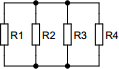# Parallel resistance calculator

## Calculates properties of resistors connected in parallel

 Example 1: Must calculate the resistance of 1Mohm, 150kohm and 47kohm resistors connected in parallel View example
 Voltage volts Resistor R1 ohms Resistor R2 ohms Resistor R3 ohms Resistor R4 ohmsYou may use one of the following SI prefix after a value: p=pico, n=nano, u=micro, m=milli, k=kilo, M=mega, G=giga

Fill at least one of the following
• Voltage - Applied voltage through all of the resistors
• R1...R4 - Resistors 1 to 4